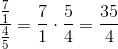## Example Questions

### Example Question #51 : Fractions

Car A traveled 120 miles with 5 gallons of fuel.

Car B can travel 25 miles per gallon of fuel.

Quantity A: The fuel efficiency of car A

Quantity B: The fuel efficiency of car B

Quantity A is greater.

The two quantities are equal.

The relationship cannot be determined.

Quantity B is greater.

Quantity B is greater.

Explanation:

Let's make the two quantities look the same.

Quantity A: 120 miles / 5 gallons =  24 miles / gallon

Quantity B: 25 miles / gallon

Quantity B is greater.

### Example Question #52 : Fractions

Quantity A:

The-value of the equation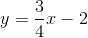when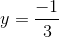Quantity B:The relationship cannot be determined from the information given.

Quantity A is greater.

Quantity B is greater.

Both quantities are equal

Quantity A is greater.

Explanation:

In order to solve quantitative comparison problems, you must first deduce whether or not the problem is actually solvable. Since this consists of finding the solution to an-coordinate on a line where nothing too complicated occurs, it will be possible.

Thus, your next step is to solve the problem.

Sinceand, you can plug in the-value and solve for:Plug in y: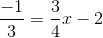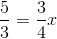Divide by 3/4.  To divide, first take the reciprocal of 3/4 (aka, flip it) to get 4/3, then multiply that by 5/3: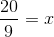Make the improper fraction a mixed number: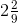Now that you have what x equals, you can compare it to Quantity B.

Sinceis bigger than 2, the answer is that Quantity A is greater

### Example Question #53 : Fractions

What is equivalent to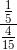?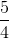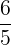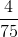Explanation:

Remember that when you divide by a fraction, you multiply by the reciprocal of that fraction.  Therefore, this division really is: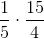At this point, it is merely a matter of simplification and finishing the multiplication: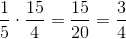### Example Question #54 : Fractions

Which of the following is equivalent to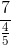?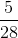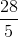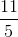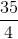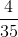Explanation:

To begin with, most students find it easy to remember that...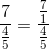From this, you can apply the rule of division of fractions.  That is, multiply by the reciprocal:

Therefore,